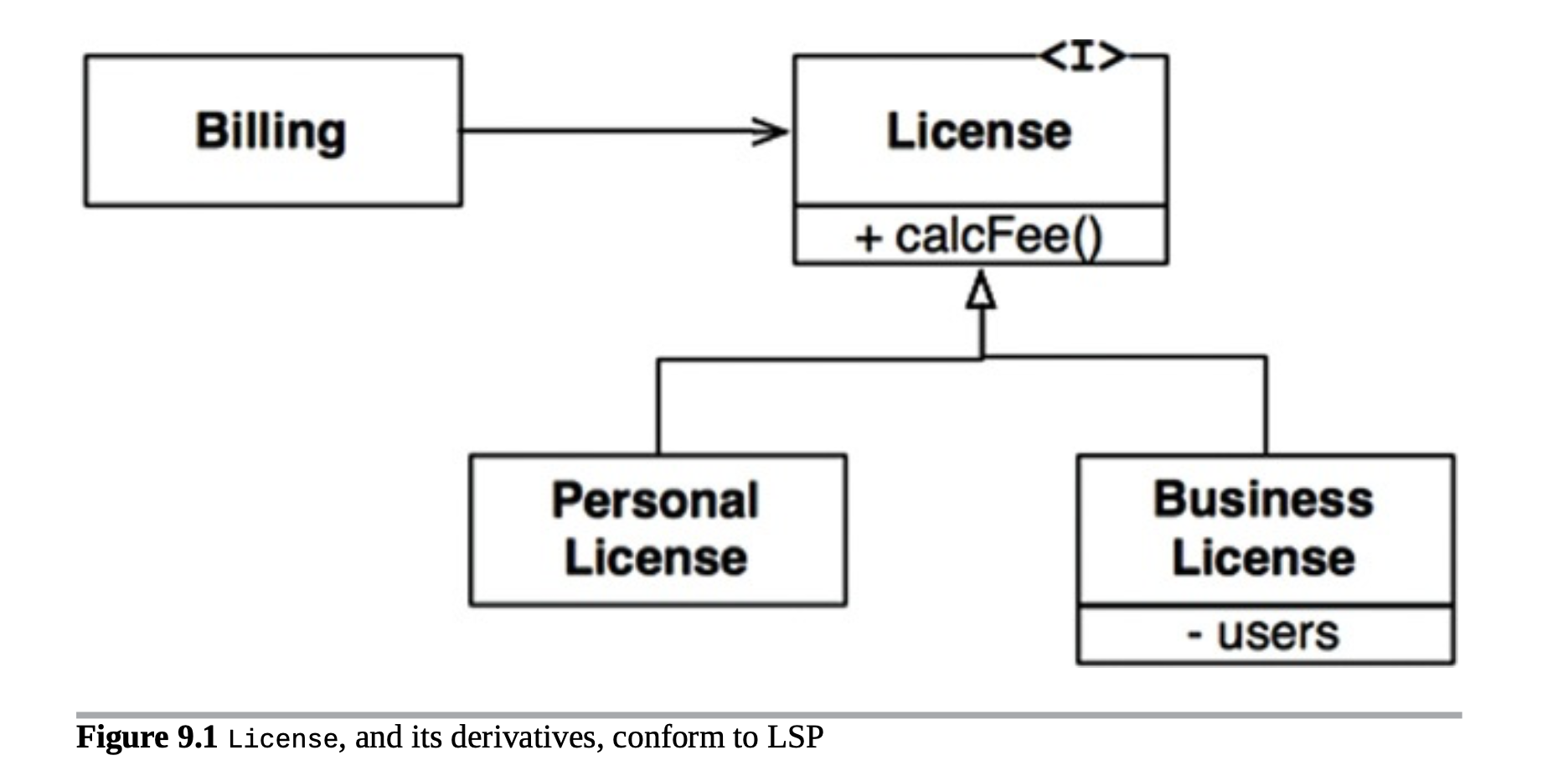# ARTS 打卡 Week 06teokingAlgorithm: 每周至少做一个 LeetCode 的算法题

Review: 阅读并点评至少一篇英文技术文章

Tips: 学习至少一个技术技巧

Share: 分享一篇有观点和思考的技术文章

﻿

# Algorithm

LC 102: Binary Tree Level Order Traversal

/* Runtime: 7 ms, faster than 6.43% of Java online submissions for Binary Tree Level Order Traversal. Memory Usage: 40.8 MB, less than 5.44% of Java online submissions for Binary Tree Level Order Traversal. */static class Solution1 {  public List<List<Integer>> levelOrder(TreeNode root) {    List<List<Integer>> answer = new ArrayList<>();    if (root == null) {      return answer;    }    Queue<TreeNode> queue = new ArrayDeque<>();    queue.offer(root);    while (queue.size() != 0) {      List<Integer> levelAnswer = new ArrayList<>();      List<TreeNode> levelNode = new ArrayList<>();      for (TreeNode node : queue) {        levelAnswer.add(node.val);        if (node.left != null) {          levelNode.add(node.left);        }        if (node.right != null) {          levelNode.add(node.right);        }      }      queue.clear();      queue.addAll(levelNode);      answer.add(levelAnswer);    }    return answer;  }}/* Runtime: 2 ms, faster than 16.72% of Java online submissions for Binary Tree Level Order Traversal. Memory Usage: 40.7 MB, less than 8.69% of Java online submissions for Binary Tree Level Order Traversal. */static class Solution2 {  public List<List<Integer>> levelOrder(TreeNode root) {    List<List<Integer>> answer = new ArrayList<>();    if (root == null) {      return answer;    }    Queue<TreeNode> queue = new ArrayDeque<>();    queue.offer(root);    // 避免queue的addAll操作    while (queue.size() != 0) {      List<Integer> levelAnswer = new ArrayList<>();      int qSize = queue.size();      for (int i = 0; i < qSize; i++) {        TreeNode tempNode = queue.poll();        levelAnswer.add(tempNode.val);        if (tempNode.left != null) {          queue.offer(tempNode.left);        }        if (tempNode.right != null) {          queue.offer(tempNode.right);        }      }      answer.add(levelAnswer);    }    return answer;  }}/* Runtime: 1 ms, faster than 60.85% of Java online submissions for Binary Tree Level Order Traversal. Memory Usage: 40.7 MB, less than 7.08% of Java online submissions for Binary Tree Level Order Traversal. */static class Solution3 {  public List<List<Integer>> levelOrder(TreeNode root) {    List<List<Integer>> answer = new ArrayList<>();    if (root == null) {      return answer;    }    // queue实现换成LinkedList    Queue<TreeNode> queue = new LinkedList<>();    queue.offer(root);    while (queue.size() != 0) {      List<Integer> levelAnswer = new ArrayList<>();      int qSize = queue.size();      for (int i = 0; i < qSize; i++) {        TreeNode tempNode = queue.poll();        levelAnswer.add(tempNode.val);        if (tempNode.left != null) {          queue.offer(tempNode.left);        }        if (tempNode.right != null) {          queue.offer(tempNode.right);        }      }      answer.add(levelAnswer);    }    return answer;  }}

﻿

# Review

https://developer.apple.com/documentation/storekit/in-app_purchase?language=objc

# Tips

1. 凡是变化的界面数值，都封装成方法

2. 以iPhone 6P为基准，其余设备的界面布局部分数值都是6P数据乘以屏幕宽度比例，从而实现按比例缩放

#define scaleWidthRatio      UIScreen.mainScreen.bounds.size.width / 375.0- (CGFloat)getBannerHeight {    return 280 * scaleWidthRatio;}

- (CGFloat)getFeatureViewBottom {    if ([Utility iPhoneX]) {        return -60.0;    } else if (IS_SCREEN_WIDTH_414()) {        return -21.0;    } else {        return -20.0;    }}

﻿

# Share

《程序员修炼之道-第二版》优秀设计精髓一章阅读感悟：ETC与设计原则（模式）的关系是什么？

﻿

﻿﻿

﻿

﻿

﻿### teoking

Monkey plays software. 2018.11.28 加入

## 评论